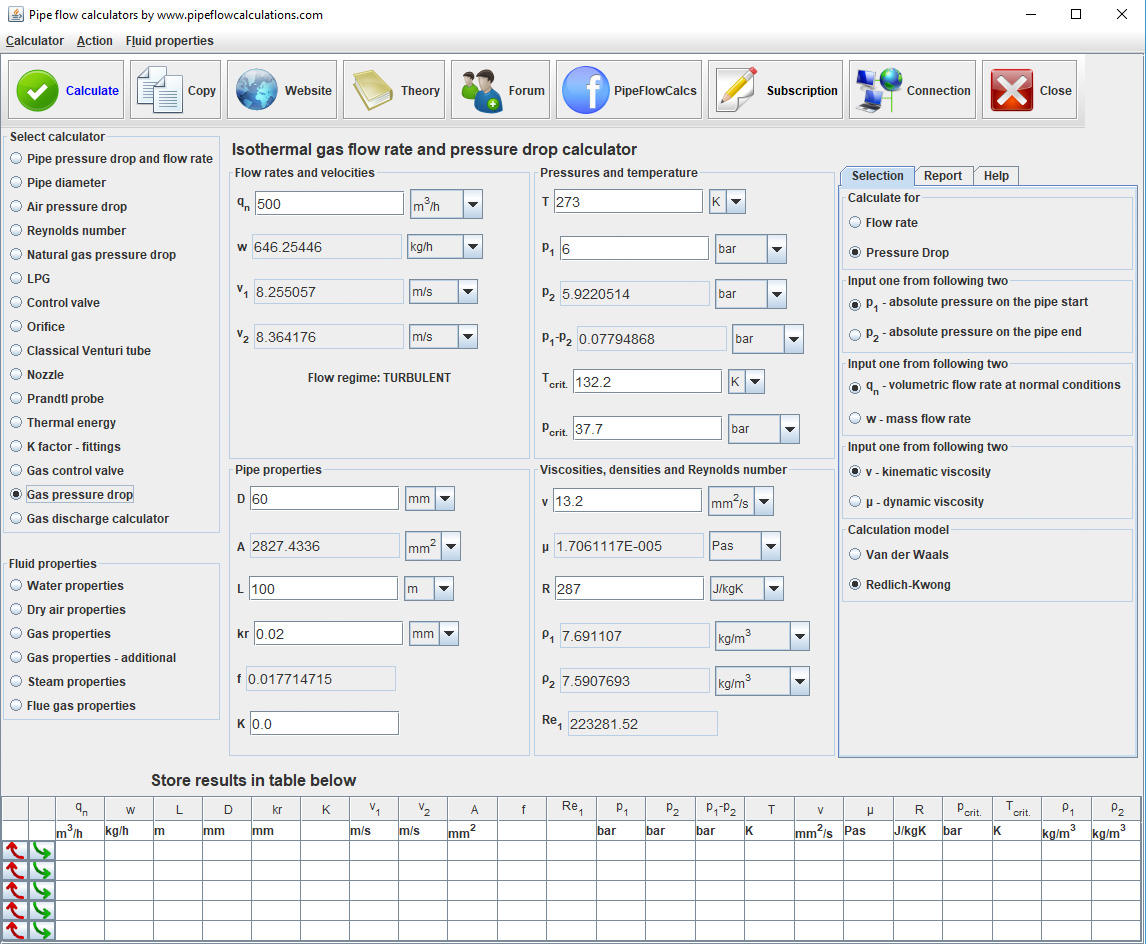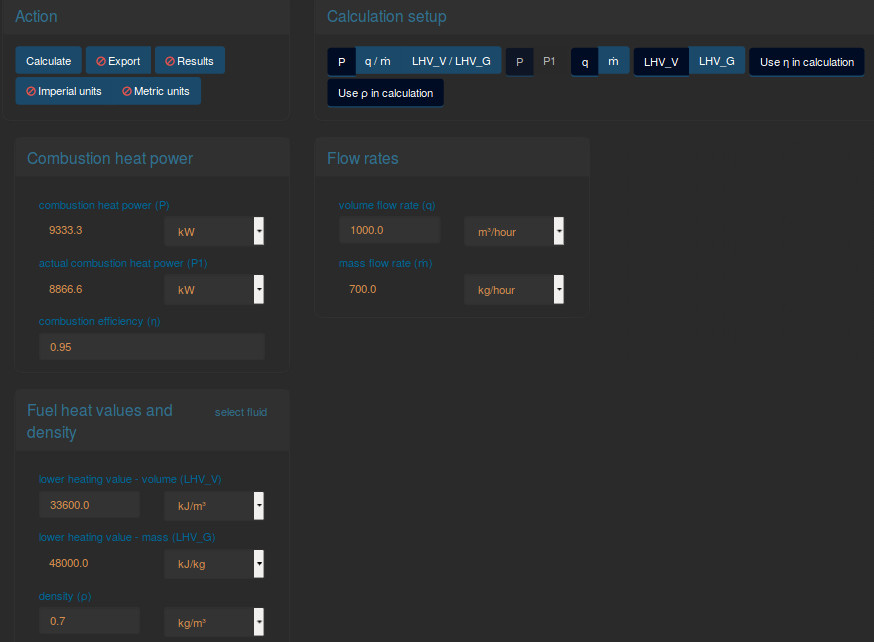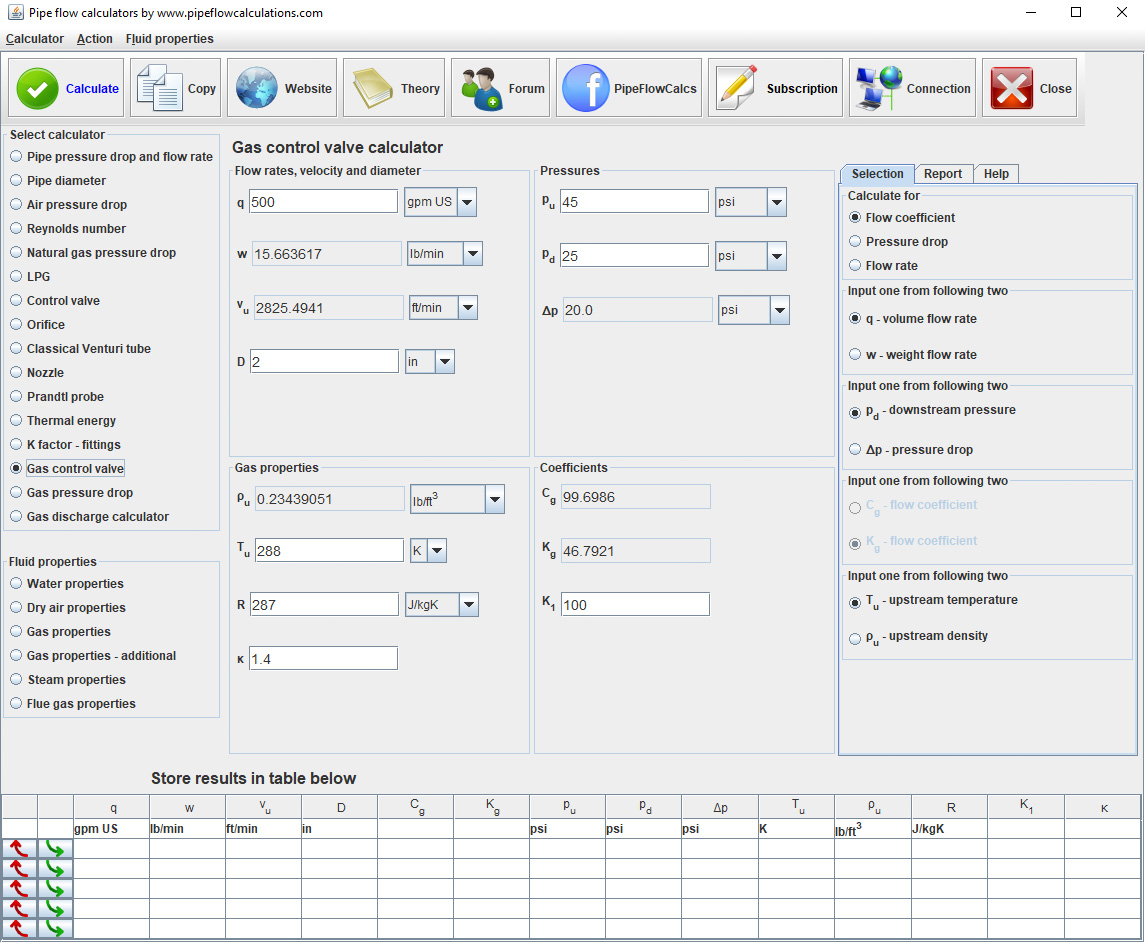LPG calculator

liquefied petroleum gas - propane/butane - pipe size, heat power

online since 2007Registration and subscription

Please login for full calculator service. Use the form on the top of the page.
Register an account if you don't have one.
Active subscription is required for full services.

save/open results
print results
custom fluid properties
K factor for fittings, resistance coefficient
compressible isothermal flow
Action
Calculation setup
LPG components ratio
Liquid phase flow
Gas phase flow
Mass flow rate and heat power
LPG gas phase properties

Need a helping hand?If you need a quick calculation, but you are not still familiar how to use the calculator, you can order calculation service from the calculator developer.

Calculator description

Read more about calculator application and check if calculator is suitable for your problem.

Also, read about calculation procedures, what you have to know for the calculations and what limitations apply.

Registration and subscription

Subscribed users have access to the full service which includes:

• One click switch from metric to imperial units
• Export results in word or excel format
• Preview, copy and paste results from a web page to your text editor
• Send results to you email

Calculator start selection

Read all about available deployments. In any way of utilizing calculator, Internet connection is required, for authentication at least.

Having problems with calculators usage? Read more about known issues and problems and way to solve it.

LPG composition

Select volume or mass percent composition
Volume fraction %vol
Ratio of propane or butane volume to the total volume of the mixture
Mass fraction %wt
Ratio of propane or butane mass to the total mass of the mixture

Description

q liq - volume flow rate of the liquid phase
LPG flow when it is in the liquid phase, before the evaporator
q gas - volume flow rate of the gas phase at normal conditions
LPG flow when it is in the gas phase, after the evaporator
V liq - velocity of the liquid phase
The velocity of LPG when it is in a liquid phase before it evaporates
V gas - velocity of the gas phase
The velocity of LPG when it is in the gas phase, after the evaporator
D liq - pipe diameter of the liquid phase
Internal pipe diameter of the LPG line liquid phase before the evaporator
D gas - pipe diameter of the gas phase
Internal pipe diameter of the LPG line gas phase after the evaporator
ṁ - mass flow rate
LPG flow rate in terms of mass flow in the unit of time. Conserved between liquid and gas phase of LPG
P - combustion heat power
Heat power generated after the combustion of LPG. Actual heat power should be reduced for the efficiency loses
LHV_G - lower heating value - mass
The heating value of LPG mixture - energy released during the combustion of a unit of mass
p - absolute pressure of LPG gas
The pressure of the LPG after the evaporator or after the pressure control valve
T - gas temperature
The temperature needed to calculate LPG density after the evaporator or after the pressure control valve
ρ gas - gas actual density
Calculated density for entered pressure and temperature, and mixture components ratio
ρ gas_nor - gas density at normal conditions
A density of LPG mixture at normal conditions p=101325 Pa and t=00C
M -molar mass
Calculated molar mass for the selected ratio of propane and butane in the LPG mixture

Calculation setup

Select value to calculate. You should enter not selected one.
P
combustion heat power calculation
q liq/q gas/ṁ
LPG flow rate calculation
Select value to input. You should enter selected one. The other two will be calculated
mass flow rate
q liq
liquid phase flow rate
q gas
gas phase flow rate
Select value to input. You should enter selected one. The other one will be calculated. Available only if the gas is selected fluid
V liq
liquid phase velocity
D liq
liquid phase pipeline diameter
Select value to input. You should enter selected one. The other one will be calculated. Available only if the gas is selected fluid
V gas
gas phase velocity
D gas
gas phase pipeline diameter